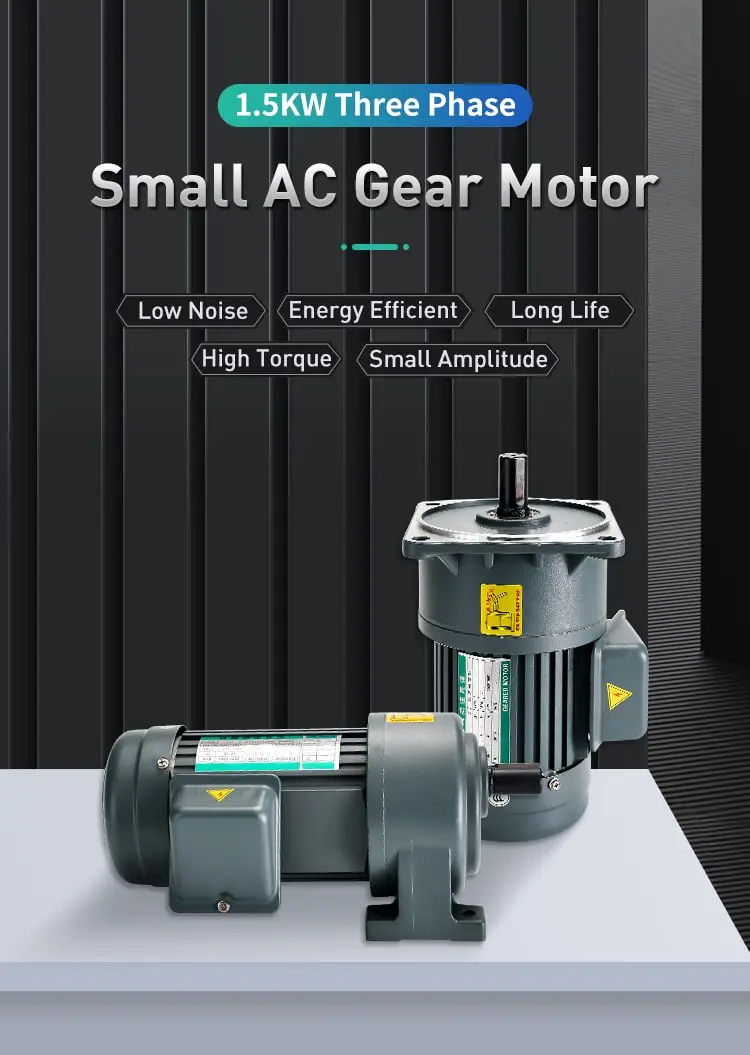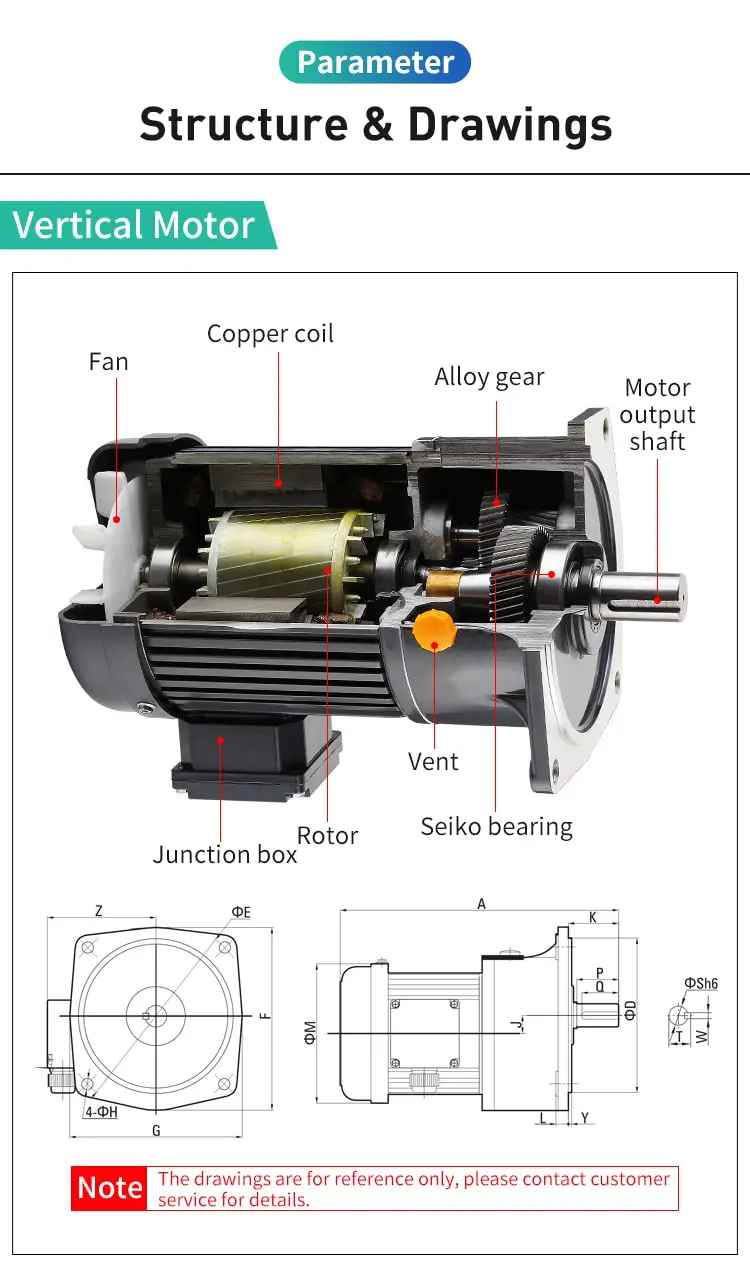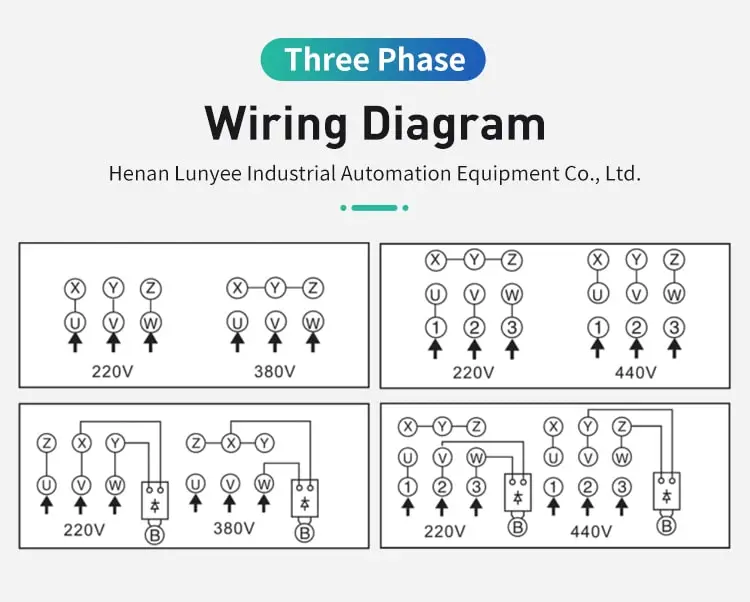By Jasmine 2023-03-07 08:49:57

#### How to calculate stator resistance of induction motor?

The stator resistance of an induction motor can be calculated by measuring the voltage drop across the stator winding and the current flowing through it. The resistance can then be calculated by dividing the voltage drop by the current.

The stator resistance of an induction motor can be calculated using the following formula:

Stator Resistance (R) = (Voltage Drop / Current)

To calculate the stator resistance of an induction motor , you will need to measure the voltage drop across the stator windings and the current flowing through the stator windings. The voltage drop across the stator windings can be measured using a voltmeter, and the current flowing through the stator windings can be measured using an ammeter.

Once you have measured the voltage drop and the current flowing through the stator windings, you can use the above formula to calculate the stator resistance of the motor. For example, if the voltage drop across the stator windings is 100 volts and the current flowing through the stator windings is 10 amps, then the stator resistance of the motor would be calculated as follows:

Stator Resistance (R) = (100 volts / 10 amps) = 10 ohms

In this example, the stator resistance of the induction motor is 10 ohms, which means that a voltage drop of 100 volts is produced across the stator windings when a current of 10 amps is flowing through the stator windings.

In summary, the stator resistance of an induction motor can be calculated using the formula: Stator Resistance (R) = (Voltage Drop / Current). To calculate the stator resistance of an induction motor, you will need to measure the voltage drop and the current flowing through the stator windings, and then use the above formula to calculate the stator resistance of the motor.